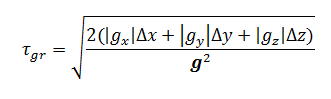## Time step in FlowVision. How to specify - Why do we need gravitational time step?

### Article Index

#### why do we need gravitational time step? and why doesn't it have a criterion?

The gravitational time step is set for the projects where the gravity vector is specified. The presence of gravity can accelerate the body or slow it down significantly. Accordingly this can increase or decrease the time step. To limit the surface and convective explicit steps from above, the gravitational step is calculated.

It is calculated by formula:Where:

• g - gravity vector;
• gcomponent of gravity vector;
• Δi - minimal cell size.